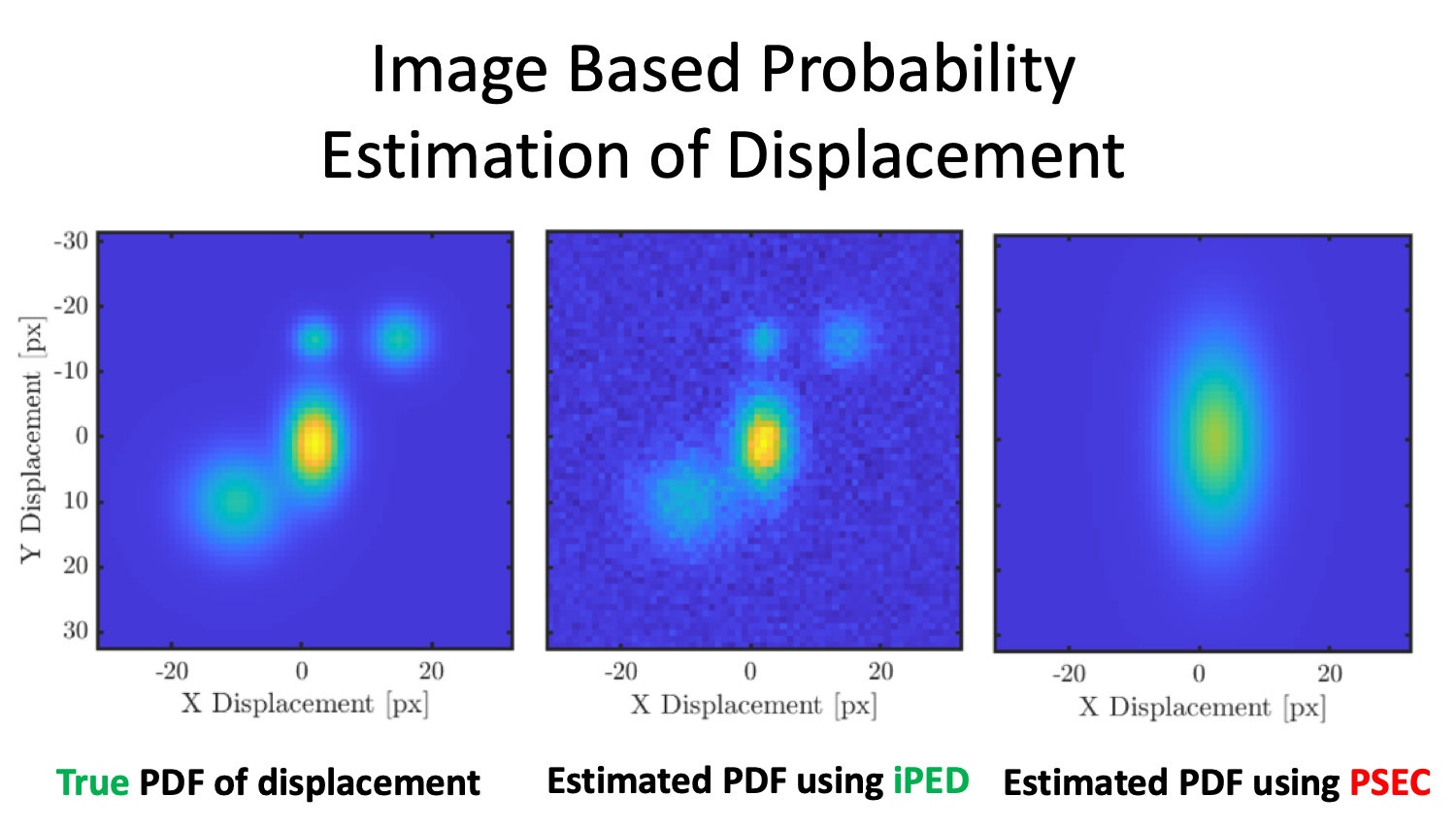Measurement of the probability density function of random motion of particle images
16697## 29 Apr Measurement of the probability density function of random motion of particle images

Estimating the probability density function (PDF) of the random motion of particles has many applications. For example, measuring diffusion, rheology, temperature, Reynolds stresses in turbulent flows, and uncertainty in velocity measurements. Current methods based on cross-correlation (CC) of image ensembles estimate an assumed Gaussian PDF’s standard deviation from the CC peak width, assuming that the particle intensity profile and distribution of particle displacements are Gaussian. In many applications, these assumptions do not hold and can lead to inaccurate estimation of the PDF. These methods are referred to as Probability Spread Estimation from Cross-correlation (PSEC)

We introduce a new method for Image-based Probability Estimation of Displacement (iPED) that does not make assumptions about the shape of particles intensity profile or the PDF of the displacements. iPED is based on the deconvolution of the Autocorrelation (AC) from the CC. We validated iPED using synthetic images and showed that it more accurately resolved the PDF of Gaussian and non-Gaussian particle shapes and processes. Finally, we apply iPED to experimental data for diffusion measurements and show significant accuracy improvements.

Figure 1. iPED algorithm for finding the PDF of displacement using deconvolution of the AC from CC.

Figure 2 (a) Simulated PDF of displacement green bar chart. iPED’s estimation of the PDF is shown as red lines, and PSEC are shown in blue for gaussian and non-gaussian particles. (b) iPED and PSEC were used for finding the diffusion coefficient of particles with different sizes compared to the expected diffusion coefficient value.